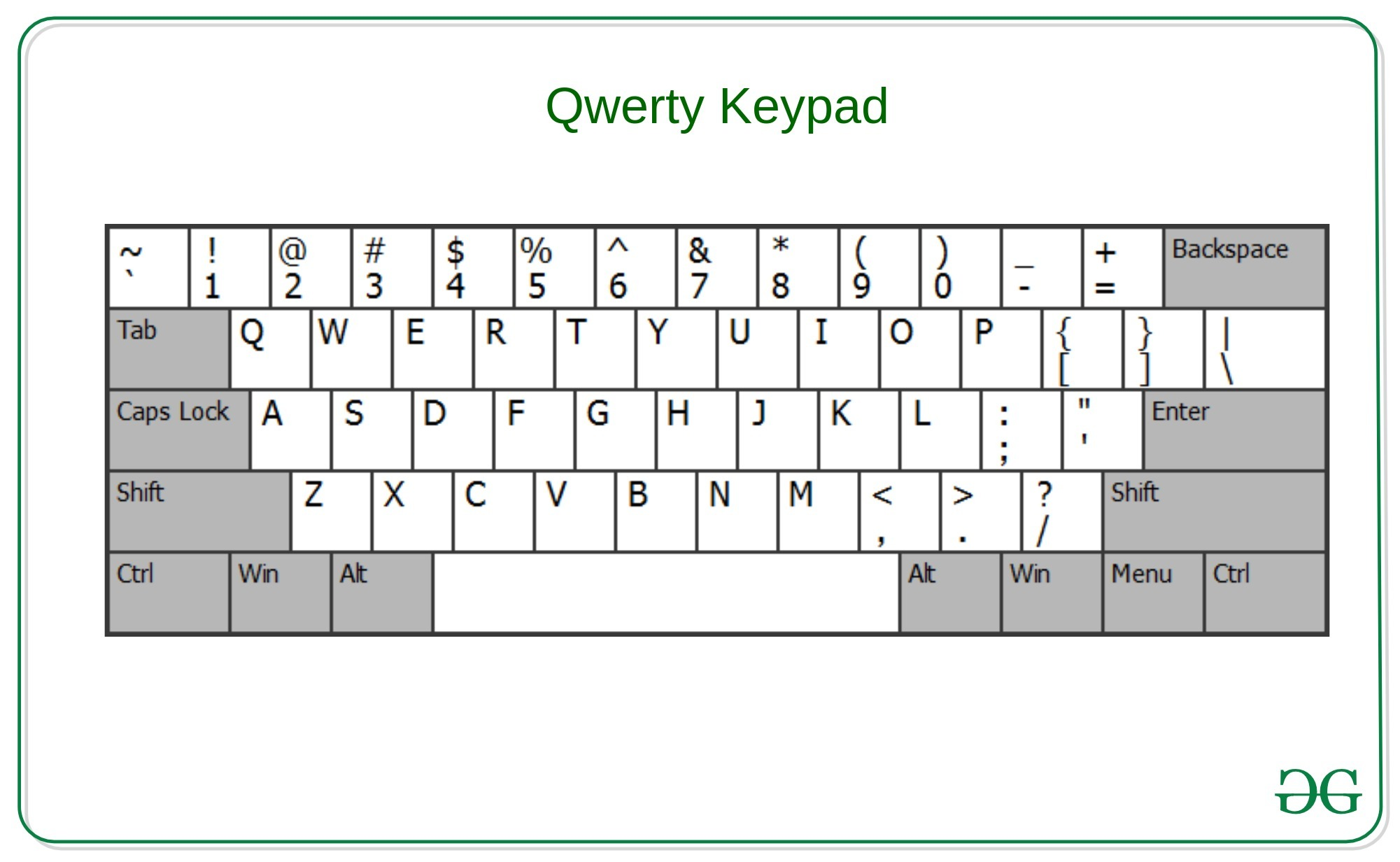# Check whether the string can be printed using same row of qwerty keypad

Given a string S, the task is to check whether the string can be typed using only a single row of the qwerty keyboard.Examples:

Output: Yes
Explanation:
Characters “D” and “a” are present in the same row of qwerty keypad. That is second Row.

Input: S = “Mom”
Output: No
Explanation:
Characters “M” and “o” are not present in the same row of qwerty keypad.

Approach: The idea is to store the characters of the same row of the qwerty keypad into different hash-maps to check that all the characters of the string are from the same row.

Below is the implementation of the above approach:

## C++

 `// C++ Program to check whether ` `// the string can be printed ` `// using same row of qwerty keypad ` ` `  `#include ` `using` `namespace` `std; ` ` `  `// Function to find the row of the ` `// character in the qwerty keypad ` `int` `checkQwertyRow(``char` `x) ` `{ ` `    ``// Sets to include the characters ` `    ``// from the same row of the qwerty keypad ` `    ``set<``char``> first_row ` `        ``= { ``'1'``, ``'2'``, ``'3'``, ``'4'``, ` `            ``'5'``, ``'6'``, ``'7'``, ``'8'``, ` `            ``'9'``, ``'0'``, ``'-'``, ``'='` `}; ` `    ``set<``char``> second_row ` `        ``= { ``'Q'``, ``'W'``, ``'E'``, ``'R'``, ``'T'``, ` `            ``'Y'``, ``'U'``, ``'I'``, ``'O'``, ``'P'``, ` `            ``'['``, ``']'``, ``'q'``, ``'w'``, ``'e'``, ` `            ``'r'``, ``'t'``, ``'y'``, ``'u'``, ``'i'``, ` `            ``'o'``, ``'p'` `}; ` `    ``set<``char``> third_row ` `        ``= { ``'A'``, ``'S'``, ``'D'``, ``'F'``, ``'G'``, ` `            ``'H'``, ``'J'``, ``'K'``, ``'L'``, ``';'``, ` `            ``':'``, ``'a'``, ``'s'``, ``'d'``, ``'f'``, ` `            ``'g'``, ``'h'``, ``'j'``, ``'k'``, ``'l'` `}; ` `    ``set<``char``> fourth_row ` `        ``= { ``'Z'``, ``'X'``, ``'C'``, ``'V'``, ``'B'``, ` `            ``'N'``, ``'M'``, ``','``, ``'.'``, ` `            ``'/'``, ``'z'``, ``'x'``, ``'c'``, ``'v'``, ` `            ``'b'``, ``'n'``, ``'m'` `}; ` ` `  `    ``// Condition to check the row of the ` `    ``// current character of the string ` `    ``if` `(first_row.count(x) > 0) { ` `        ``return` `1; ` `    ``} ` `    ``else` `if` `(second_row.count(x) > 0) { ` `        ``return` `2; ` `    ``} ` `    ``else` `if` `(third_row.count(x) > 0) { ` `        ``return` `3; ` `    ``} ` `    ``else` `if` `(fourth_row.count(x) > 0) { ` `        ``return` `4; ` `    ``} ` ` `  `    ``return` `0; ` `} ` ` `  `// Function to check the characters are ` `// from the same row of the qwerty keypad ` `bool` `checkValidity(string str) ` `{ ` `    ``char` `x = str; ` `    ``int` `row = checkQwertyRow(x); ` `    ``for` `(``int` `i = 0; i < str.length(); i++) { ` `        ``x = str[i]; ` `        ``if` `(row != checkQwertyRow(x)) { ` `            ``return` `false``; ` `        ``} ` `    ``} ` `    ``return` `true``; ` `} ` ` `  `// Driver Code ` `int` `main() ` `{ ` `    ``string str = ``"GeeksforGeeks"``; ` ` `  `    ``if` `(checkValidity(str)) ` `        ``cout << ``"Yes"``; ` `    ``else` `        ``cout << ``"No"``; ` ` `  `    ``return` `(0); ` `} `

Output:

```No
```

Time Complexity: O(N)

Don’t stop now and take your learning to the next level. Learn all the important concepts of Data Structures and Algorithms with the help of the most trusted course: DSA Self Paced. Become industry ready at a student-friendly price.

My Personal Notes arrow_drop_upCheck out this Author's contributed articles.

If you like GeeksforGeeks and would like to contribute, you can also write an article using contribute.geeksforgeeks.org or mail your article to contribute@geeksforgeeks.org. See your article appearing on the GeeksforGeeks main page and help other Geeks.

Please Improve this article if you find anything incorrect by clicking on the "Improve Article" button below.